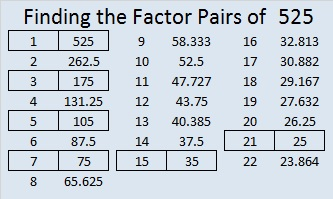# 525 and Level 3

525 = (23 + 2)(23 – 2) = (23^2) – (2^2) makes 525 the longer leg in what primitive Pythagorean triple?

Why do people enjoy number puzzles? A mathemagician friend once stated that a key reason was loving to solve mysteries.

I find making and solving puzzles to be quite relaxing. Solve the mystery or relax a little finding the factors of this puzzle:Print the puzzles or type the solution on this excel file: 12 Factors 2015-06-15

—————————————————————————————————

• 525 is a composite number.
• Prime factorization: 525 = 3 x 5 x 5 x 7, which can be written 525 = 2 x (5^2) x 7
• The exponents in the prime factorization are 1, 2, and 1. Adding one to each and multiplying we get (1 + 1)(2 + 1)(1 + 1) = 2 x 3 x 2 = 12. Therefore 525 has exactly 12 factors.
• Factors of 525: 1, 3, 5, 7, 15, 21, 25, 35, 75, 105, 175, 525
• Factor pairs: 525 = 1 x 525, 3 x 175, 5 x 105, 7 x 75, 15 x 35, or 21 x 25
• Taking the factor pair with the largest square number factor, we get √525 = (√25)(√21) = 5√21 ≈ 22.912878—————————————————————————————————

A Logical Approach to solve a FIND THE FACTORS puzzle: Find the column or row with two clues and find their common factor. Write the corresponding factors in the factor column (1st column) and factor row (top row).  Because this is a level three puzzle, you have now written a factor at the top of the factor column. Continue to work from the top of the factor column to the bottom, finding factors and filling in the factor column and the factor row one cell at a time as you go.## One thought on “525 and Level 3”

1.abyssbrain

Thank you for linking to my post!

This site uses Akismet to reduce spam. Learn how your comment data is processed.